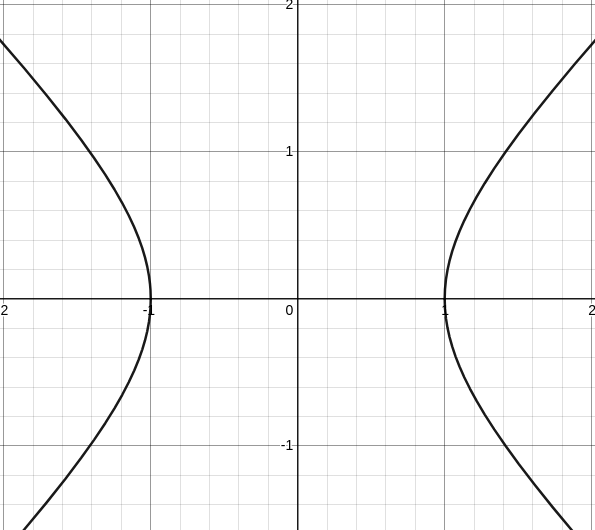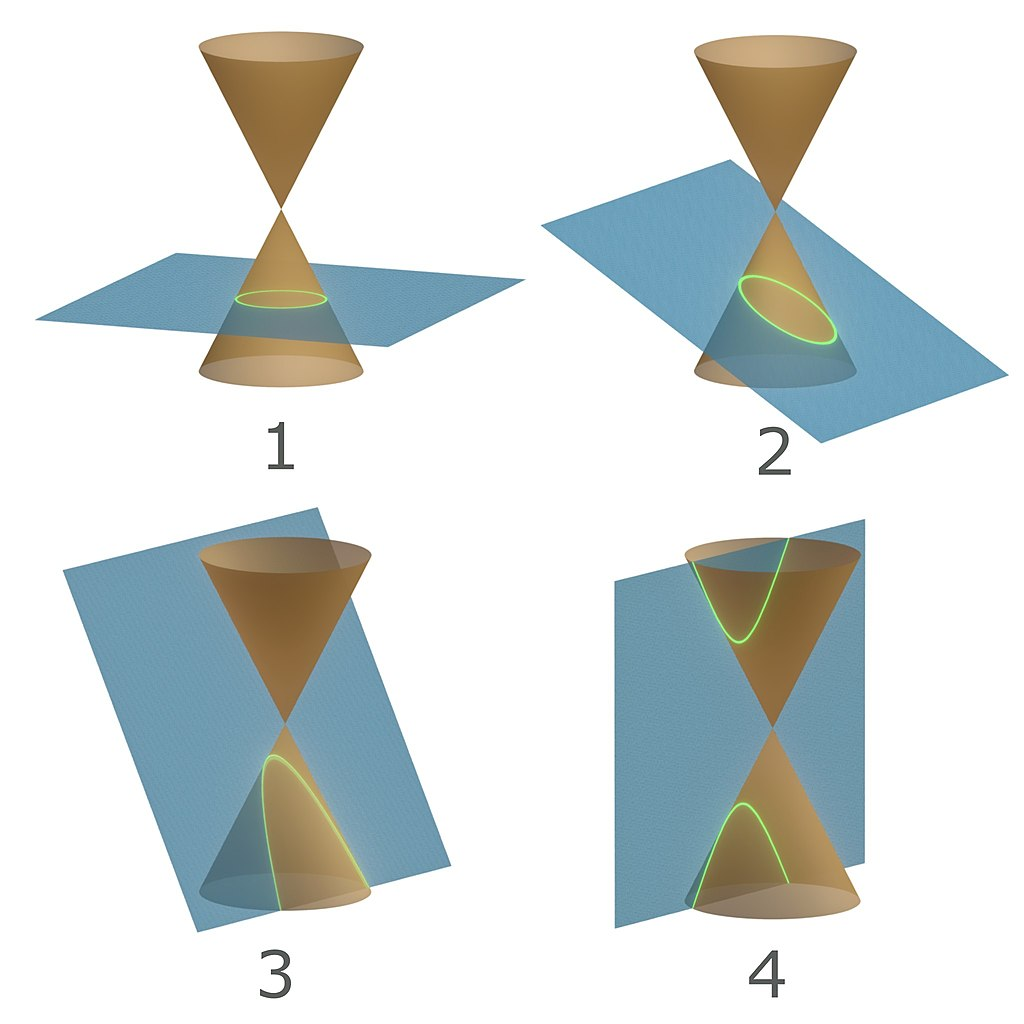# Hyperbolics

Created 2021-09-11, Edited 2022-03-12
Deriving cosh and sinh from first principles

Hyperbolas are great, they're used to describe gravity assists, the shape power lines or chains make when hanging off two points is a Catenary.

This post is about the hyperbolic functions $\cosh(t), \sinh(t)$ that describe hyperbolics parametrically. In particular I show how you might have discovered them yourself!This is the unit hyperbola, described by $x^2 - y^2 = 1$. We want to find $x(t), y(t)$ that describe this curve. Since they are always on the curve they must satisfy

$x(t)^2 - y(t)^2 = 1$

Knowing how $x(t),y(t)$ change will help us find $x(t)$ and $y(t)$, so let's differentiate using implicit differentiation

$2x(t)x'(t) - 2y(t)y'(t) = 0 \implies x(t)x'(t) = y(t)y'(t) \implies \frac{x}{y} = \frac{y'}{x'}$

Hey! That means $x' = Cy$ and $y' = Cx$ for some constant $C$ (since rational numbers are unique up to a constant multiple) This reminds me of $e^{Ct}$, except there are two functions in a cycle instead of one.

We can use this to find all the derivatives in terms of $x$ and $y$

$x' = Cy \implies x'' = Cy' = C^2 x$

Hey! If differentiating $x$ gives us $Cy$ and vise versa, then differentiating $x(t) + y(t)$ should stay the same up to a multiple of $C$, Let's do this

$\frac{d}{dt}\left[x(t) + y(t)\right] = Cy(t) + Cx(t) = C(x(t) + y(t))$

If we denote $s(t)$ to be the sum $x(t) + y(t)$ then we have $s'(t) = Cs(t)$.

This reminds me of $e^{Ct}$ since it's derivative is $C$ times itself, so I'm going to write $s(t)$ as a Maclaurin Series and try to find $s(t)$ in terms of $e^{Ct}$, but first we need to decide what $s(0)$ is, let's look at the graph againWhere should our parametric equations start? All points work fine, but we'll pick $(1, 0)$ for two reasons

1. It's a natural seeming starting point
2. Having $x(0) = 1, y(0) = 0$ will drastically simplify our maclaurin series

Now that we have our starting point, we can find the maclaurin series of $s(t)$

\begin{aligned} s(t) &= \sum_{k=0}^{\infty} \frac{s^{(n)}(0)t^k}{k!} \\ &= \sum_{k=0}^{\infty} \frac{(Ct)^k}{k!} \\ \end{aligned}

You might recognize this series, it's the series for $e^{Ct}$!, therefor $s(t) = e^{Ct}$

Now I want to find $x(t)$ and $y(t)$ individually, going back to the original equation I notice it's a difference of squares, meaning we can factor it as

$(x - y)(x + y) = 1 \implies (x - y)e^{Ct} = 1 \implies x-y = e^{-Ct}$

Adding the sum and difference we can recover the individual equations!

\begin{aligned} x(t) &= \frac{(x + y) + (x - y)}{2} = \frac{e^{Ct} + e^{-Ct}}{2} \\ y(t) &= \frac{(x + y) - (x - y)}{2} = \frac{e^{Ct} - e^{-Ct}}{2} \end{aligned}

Let's check our work, our functions should satisfy $x(t)^2 - y(t)^2 = 1$

\begin{aligned} &\frac{1}{4}\left( (e^{Ct} + e^{-Ct})^2 - (e^{Ct} - e^{-Ct})^2 \right) \\ = &\frac 14\left( (e^{2Ct} + 2\bcancel{e^{Ct}e^{-Ct}} + e^{-2Ct}) - (e^{2Ct} - 2\bcancel{e^{Ct}e^{-Ct}} + e^{-2Ct}) \right) \\ = &\frac 14\left( (e^{2Ct} + 2 + e^{-2Ct}) - (e^{2Ct} - 2 + e^{-2Ct}) \right) \\ = &\frac 14(4) = 1 \end{aligned}

Great! our $x(t)$ and $y(t)$ work, and they are $\cosh(t)$ and $\sinh(t)$ when $C=1$.

## Arc length reparameterization

Picking $C=1$ seems like an arbitrary choice, let's try to reparameterize in terms of arc length to give $C$ geometric meaning

\begin{aligned} s &= \int \sqrt{x'(t)^2 + y'(t)^2}dt \\ &= C \int \sqrt{y(t)^2 + x(t)^2}dt \\ &= C \int \sqrt{\cosh(t)^2 + \sinh(t)^2}dt \end{aligned}

You can try to evaluate this integral, but you can't! this is a Nonelementary integral (see wolframalpha).

## Relation to other conics

If you noticed the similarity between $\cosh, \sinh$ (for describing hyperbolas) and $\cos, \sin$ (for describing circles and ellipses) You might be wondering about expressing other conics parametrically.

The only other conic is a parabola (as circles are a special case of an ellipse). parabolas are described by $y = ax^2$, which is already a function!

Visually parabolas (3) look different from the other conics, they just barely hug the coneI like to think of this in terms of their Eccentricity

• Ellipses have eccentricity less then one
• Parabolas have eccentricity equal to one
• Hyperbolas have eccentriciy greater then one

Parabolas really are the special child in the family! They are the only conic with a single eccentricity. Here's a desmos graph showing this off

I plan to write about parabolas some time, since they show up everywhere in algebra I want to become more acquainted with their amazing properties